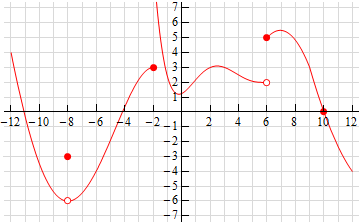Paul's Online Notes
Home / Calculus I / Limits / Continuity
Show Mobile Notice Show All Notes Hide All Notes
Mobile Notice
You appear to be on a device with a "narrow" screen width (i.e. you are probably on a mobile phone). Due to the nature of the mathematics on this site it is best views in landscape mode. If your device is not in landscape mode many of the equations will run off the side of your device (should be able to scroll to see them) and some of the menu items will be cut off due to the narrow screen width.

### Section 2.9 : Continuity

2. The graph of $$f\left( x \right)$$ is given below. Based on this graph determine where the function is discontinuous.Show Solution

Before starting the solution recall that in order for a function to be continuous at $$x = a$$ both $$f\left( a \right)$$ and $$\mathop {\lim }\limits_{x \to a} f\left( x \right)$$ must exist and we must have,

$\mathop {\lim }\limits_{x \to a} f\left( x \right) = f\left( a \right)$

Using this idea it should be fairly clear where the function is not continuous.

First notice that at $$x = - 8$$ we have,

$\mathop {\lim }\limits_{x \to - {8^ - }} f\left( x \right) = - 6 = \mathop {\lim }\limits_{x \to - {8^ + }} f\left( x \right)$

and therefore, we also know that $$\mathop {\lim }\limits_{x \to - 8} f\left( x \right) = - 6$$. We can also see that $$f\left( { - 8} \right) = - 3$$ and so we have,

$- 6 = \mathop {\lim }\limits_{x \to - 8} f\left( x \right) \ne f\left( { - 8} \right) = - 3$

Because the function and limit have different values we can conclude that $$f\left( x \right)$$ is discontinuous at $$x = - 8$$.

Next let’s take a look at $$x = - 2$$ we have,

$\mathop {\lim }\limits_{x \to - {2^ - }} f\left( x \right) = 3 \ne \infty = \mathop {\lim }\limits_{x \to - {2^ + }} f\left( x \right)$

and therefore, we also know that $$\mathop {\lim }\limits_{x \to \, - 2} f\left( x \right)$$ doesn’t exist. We can therefore conclude that $$f\left( x \right)$$ is discontinuous at $$x = - 2$$ because the limit does not exist.

Finally let’s take a look at $$x = 6$$. Here we can see we have,

$\mathop {\lim }\limits_{x \to {6^ - }} f\left( x \right) = 2 \ne 5 = \mathop {\lim }\limits_{x \to {6^ + }} f\left( x \right)$

and therefore, we also know that $\mathop {\lim }\limits_{x \to \,6} f\left( x \right)$ doesn’t exist. So, once again, because the limit does not exist, we can conclude that $$f\left( x \right)$$ is discontinuous at $$x = 6$$.

All other points on this graph will have both the function and limit exist and we’ll have $$\mathop {\lim }\limits_{x \to a} f\left( x \right) = f\left( a \right)$$ and so will be continuous.

In summary then the points of discontinuity for this graph are : $$x = - 8$$, $$x = - 2$$ and $$x = 6$$.